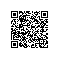# 利用index、small函数查询返回多个值=INDEX($B:$B,SMALL(IF($A$2:$A$11=$D2,ROW($A$2:$A\$11),4^8),COLUMN(A1)))&""使用钉钉扫一扫加入圈子
+ 订阅